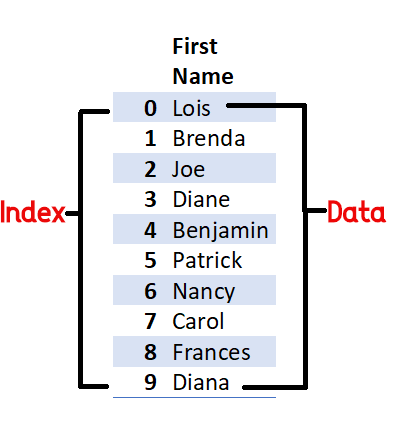Series is a one-dimensional Data Structure of Pandas, it is used for data analysis. It can contain a heterogeneous types of values. Series type Object has two main components:
• An array of actual data
• An associated array of indexes or data labelsFig-1 Series

Like list, there are indexes in Series, but in series, we can assign our own indexes (Data labels). The labels need not be unique but must be a hashable type.

## How to create pandas series?

To create the Series Object a list of data to be passed in Series() Function. This function is available inside the Pandas Module. Hence before creating Series Object we have to import pandas library using:

import pandas

Following is the syntax for Series Creation:

<Series Object> = pandas.Series(data)
<Series Object> = pandas.Series(data=None, index=None, dtype=None, name=None, copy=False)

data: data parameter provides data for Series creation, it can be a Sequence or Scalar Value
index: This is used to change the default index of Series
dtype: It is used to change the default datatype of Series
name: To give a name to Series
copy: To copy input Data, A boolean Value by default is false

By default, the index of series will be integers starting from 0,1,2...etc. as shown above in Fig-1 Series.

### Use of different types of Data

In Series Data Structure, we can use our own data for creating the series. This data can be of different types.

### <Series Object> =  pandas.Series(data=None, index=None, dtype=None, name=None, copy=False, fastpath=False)

The data can be:
• Without Data (Empty Series)
• A scalar value
• A python sequence (ex:- list, tuple, dictionary etc.)
• A ndarray
Let us discuss each one by one with Pandas Series Examples.

#### a) Without Data (Empty Series)

If we do not provide any data then python pandas will create an empty series. The default data type that pandas provide to series is "float64". In the following example, you can see that I have not provided any parameter in Series() function. This will create an empty series.
```import pandas as pd
s = pd.Series()
print(s)         ## Series([], dtype: float64)
print(type(s))   ## <class 'pandas.core.series.Series'>
```

#### b) A Scalar Value

A Pandas Series can be created with only single Parameters. This will create a Series Object with only a single value. In the following example, you can see that I have provided a value 20 only. A Series Object 's' has been created by Pandas with value 20.
```import pandas as pd
s = pd.Series(20)
print(s)
print(type(s))

'''
## Output
-----------
0    20
dtype: int64

<class 'pandas.core.series.Series'>
'''
```

#### c) A python sequence (ex:- list, tuple, dictionary etc.)

A Python sequence can be used for the creation of Series Object. Any Python Sequence like list, tuple or dictionary can be used for this purpose. Next, we will create a series using List and Dictionary.

Using List: When we use list fr creation of Series, it takes the index of Series same as the index of the list. As shown in the example the list myList is shaving Three values, these values can be of any type.
```import pandas as pd
myList = ['A','B',2]
s = pd.Series(myList)
print(s)
print(type(s))

'''
Output:
-------
0    A
1    B
2    2
dtype: object

<class 'pandas.core.series.Series'>
'''
```

Using Dictionary: When a dictionary is used for Series creation, the values of that dictionary is used as data of Series and Keys are used as data labels (index) of Series.
```import pandas as pd
d = {'A':1, 'B':2, 'C':3}
print(d)
s = pd.Series(d)
print(s)
print(type(s))

'''
Output:
------
{'A': 1, 'B': 2, 'C': 3}

A    1
B    2
C    3
dtype: int64

<class 'pandas.core.series.Series'>
'''
```

#### d) A ndarray

Numpy is a core library for numerical and scientific computation. We can use a one-dimensional Numpy array for Series creation. The Index or data labels of Series will come as 0,1,2....etc. by default.
```import numpy as np
import pandas as pd
arr = np.array([1,2,3,4])
print(arr)
s = pd.Series(arr)
print(s)
print(type(s))

'''
Output:
-------
[1 2 3 4]

0    1
1    2
2    3
3    4
dtype: int32

<class 'pandas.core.series.Series'>
'''
```